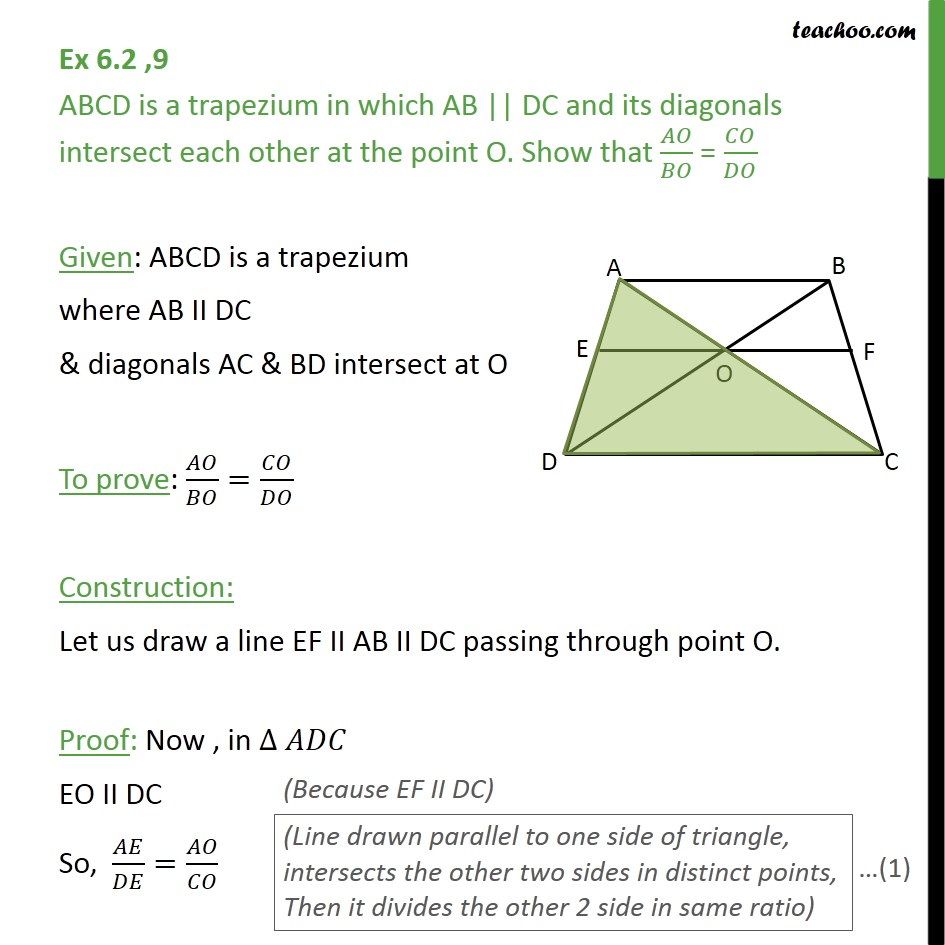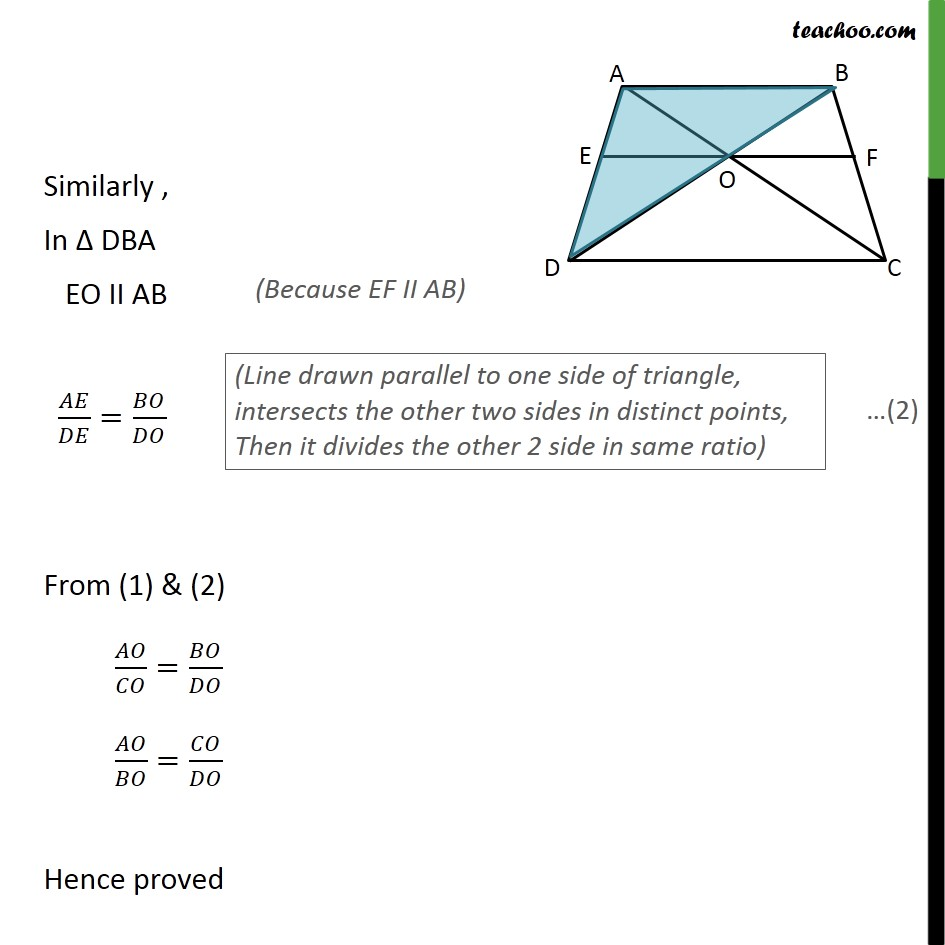Subscribe to our Youtube Channel - https://you.tube/teachoo

1. Chapter 6 Class 10 Triangles
2. Concept wise
3. Theorem 6.1

Transcript

Ex 6.2 ,9 ABCD is a trapezium in which AB || DC and its diagonals intersect each other at the point O. Show that / = / Given: ABCD is a trapezium where AB II DC & diagonals AC & BD intersect at O To prove: / = / Construction: Let us draw a line EF II AB II DC passing through point O. Proof: Now , in EO II DC So, / = / Similarly , In DBA EO II AB / = / From (1) & (2) / = / / = / Hence proved

Theorem 6.1